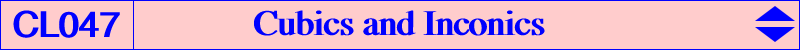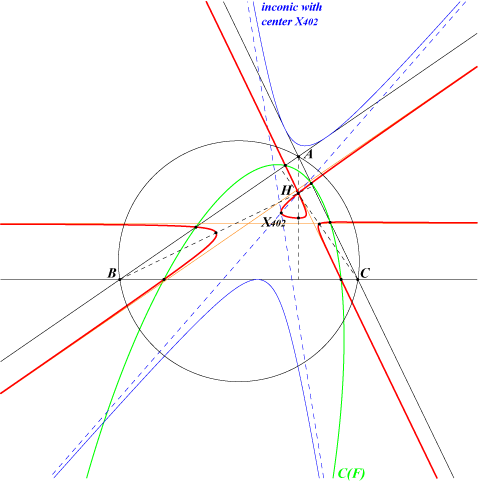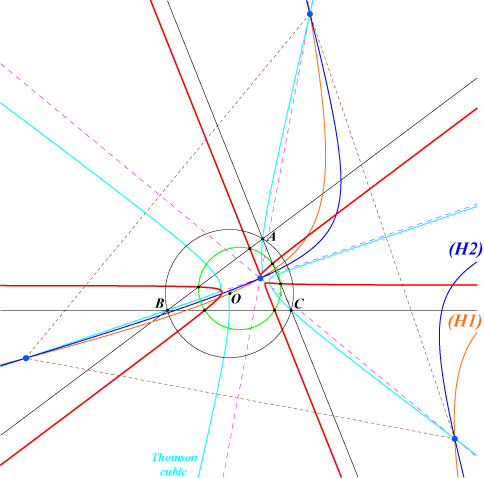In this page, we consider cubics related to inconics such that one asymptote or one axis passes through a fixed point F = u:v:w not lying on a sideline of the reference triangle ABC. See the related Allardice cubics in CL010.Cubics Cas(F) The locus of the centers of the inconics having an asymptote passing through F is the cubic Cas(F) with equation : vwx (x+y-z)(x-y+z) - u^2 (y+z-x) yz + cyclic = 0. Properties : – When F lies on the line at infinity, Cas(F) degenerates into the line at infinity an the diagonal conic with equation vwx^2 + cyclic = 0. This will be ruled out in the sequel. – Cas(F) is a nodal cubic with node F. The nodal tangents are parallel to the asymptotes of the circum-conic with perspector F^2 (barycentric square). They are perpendicular if and only if F^2 lies on the orthic axis i.e. F lies on the polar circle. – Cas(F) has three real asymptotes parallel to the sidelines of ABC. They form a triangle homothetic to ABC at the point S = u(2v+2w+u) : : , the ratio being (u^2+v^2+w^2) / [2(u+v+w)^2]. S lies on the line through F and F^2. It is the barycentric product of F and the image of F under h(G,–1/5). – Cas(F) passes through the midpoints of the cevians of F. The tangents at these points are the sidelines of the medial triangle. – Cas(F) meets the sidelines of ABC at six points lying on a same conic C(F). The triangle formed by the midpoints (one on each sideline of ABC) is perspective with ABC at the point homothetic of F under h(G,2/5).The figure shows Cas(H) passing through X(402) and the midpoints of the altitudes.   Construction of Cas(F) : the center of the inconic having an asymptote L(F) passing through F is the intersection M of L(F) and the Newton line of the quadrilateral formed by the lines BC, CA, AB, L(F). This gives an easy construction of M.The conic C(F) is a circle if and only if F is one of the four common points of the rectangular hyperbolas of a pencil containing : – the rectangular hyperbola (H1) passing through X(3), X(6), X(382), X(2574), X(2575) hence having its asymptotes parallel to those of the Jerabek hyperbola. (H1) is the polar conic of X(3) in the Neuberg cubic K001. – the anticomplement (H2) of the Steiner (or Don Wallace) hyperbola. This Steiner hyperbola is the diagonal conic passing through the in/excenters, X(2), X(20) and many other points.These four points lie on the Thomson cubic. They are the four blue points on the figure. They obviously form an orthocentric system. The cubic represented is that corresponding to the blue point lying inside the triangle ABC.Cubics Cax(F) The locus of the centers of the inconics having an axis passing through F is the cubic Cax(F) with equation : a^2(wy-vz)[-2uyz + w(x+y-z)y + v(x-y+z)z] + cyclic = 0. Properties : – When F lies on the line at infinity, Cax(F) degenerates into the line at infinity an the diagonal conic with equation a^2(w^2y^2 – v^2z^2)^2 + cyclic = 0. This will be ruled out in the sequel. – When F is an in/excenter Ix, Cax(F) degenerates into the three bisectors through Ix.This also will be ruled out in the sequel. – Cax(F) is a nodal cubic with node F. The nodal tangents are parallel to the asymptotes of the diagonal rectangular hyperbola passing through the in/excenters and F. These tangents are actually the axes of the inconic with center F. – Cax(F) contains : the in/excenters. the infinite points of pK(X6, F). It follows that Cax(F) is a K60 if and only if F = O. In this case Cax(F) = K258. the projections of F on the six bisectors of ABC. the midpoint of FF* (F* is the isogonal conjugate of F). the midpoints of the cevians of F. – Cax(F) is a K+ if and only if F lies on the cubic K077. – Cax(F) contains the center of the inconic with perspector F if and only if F lies on Q012, the Stothers quintic. See K858 = Cax(X4) for instance. *** Construction of Cax(F) : Cax(F) is the generalized Lemoine cubic K(F) of any of the four triangles with vertices three of the four in/excenters (provided that they are not collinear) and, in particular, the excentral triangle. This gives many interesting properties and several simple constructions. For example, a line L(F) through F meets the rectangular hyperbola through the in/excenters and the infinite point of L(F) at N on Cax(F). Note that if two perpendicular lines L(F) and L'(F) give the points N and N' on Cax(F), then the line NN' passes through the midpoint of FF*.